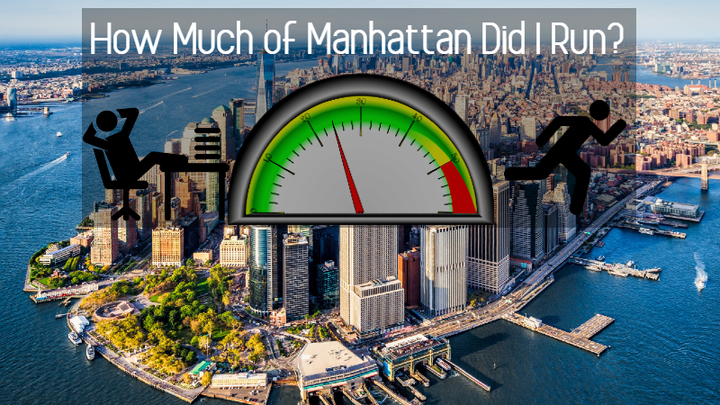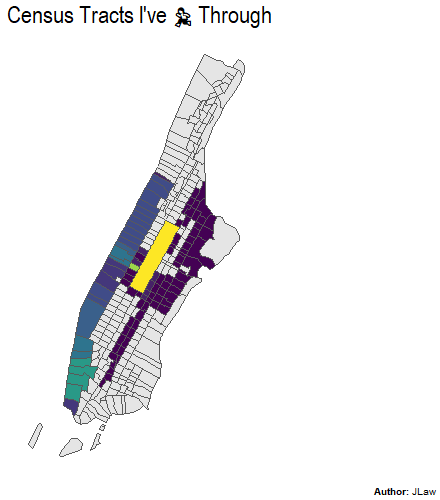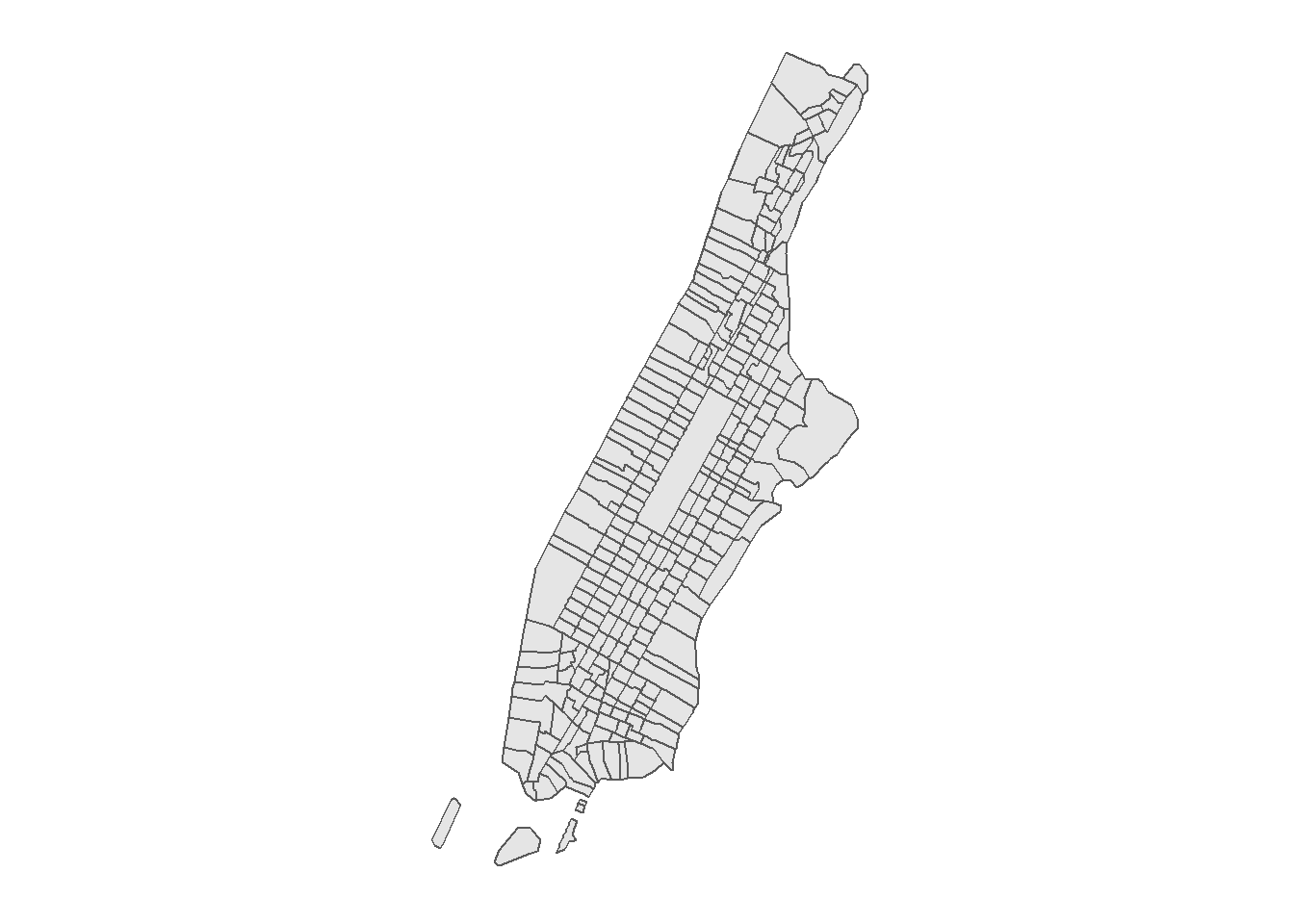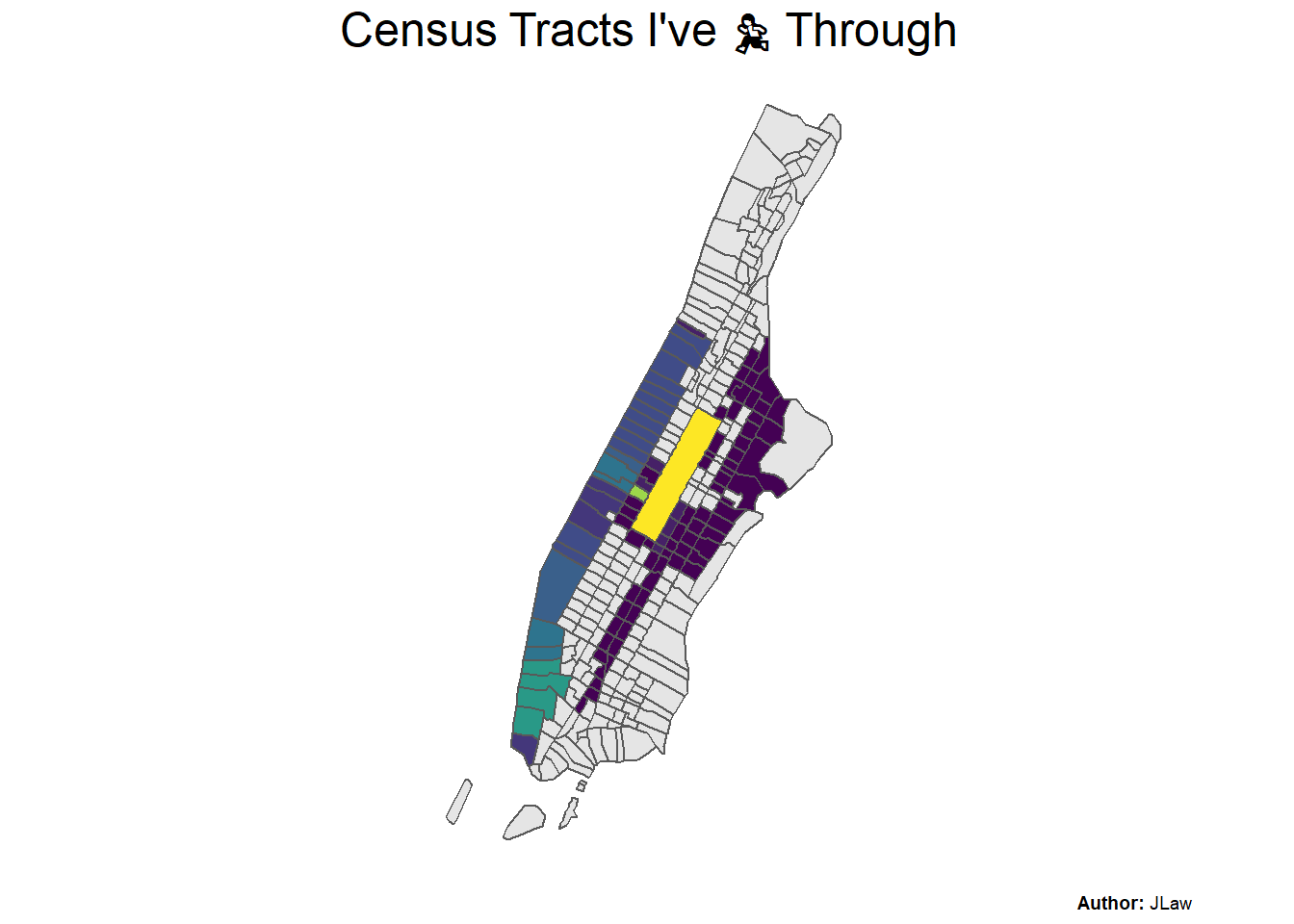# What % of Manhattan Did I Run Through?In a previous post I created a cool-looking (in my opinion) heatmap of my Marathon training from years back. One of the downsides to that density-based method of making the heat map was that routes I only ran once didn’t show up very clearly. I also wanted to know roughly what % of Manhattan I covered in my runs. This post will use that same data to create a choropleth map by Census Tract to both visualize all the tracts I passed through in my training as well as determine what % of Manhattan’s land area did I cover.

## Libraries Used

The packages used in this analysis are the same from the prior analysis, `Tidyverse` for data manipulation, `sf` for modifying spatial data, `tigris` for getting the basemaps to plot my routes and `extrafont` to bring in new fonts for the plots.

``````library(tidyverse) # Data Manipulation
library(sf) # Manipulation Spatial Data
library(tigris) # Getting Tract and Roads Spatial Data
library(extrafont) # Better Fonts For GGPLOT``````

## Data Used

The data is also the same running route data from the prior post. For more details on its creation please reference the prior post.

``````runs_and_routes <- readRDS('data/runs_and_routes.RDS')

For the basemap I’m again using the `tigris` package however this time getting census tracts rather than roads. According to the package, Census tracts generally have a population size between 1,200 and 8,000 people, with an optimum size of 4,000 people. The map is downloaded using the `tracts()` function with inputs for state and county.

``````nyc_tracts <- tracts("NY", "New York", cb = T) %>%
st_transform(crs = st_crs(runs_and_routes\$geometry))``````
``ggplot() + geom_sf(data = nyc_tracts) + ggthemes::theme_map()``Unlike the prior analysis where the heatmap was just overlaid atop the map, here I need to identify which census tracts contained a route I ran vs. which didn’t. This can be done using the `st_join` function, specifying it to be a left join, and specifying the join type as `st_intersects` which joins the route information if the lat/long is contained in the census tract. The data is then grouped by tract_name and some other tract metadata. Then I create a field for the number of routes contained in each census tract, which will be used for the choropleth.

``````#Join Routes to Tracts by Intersecting
nyc_geo_join <- nyc_tracts %>%
st_join(all_routes %>% distinct(route_id, geometry),
join = st_intersects,
left = T
) %>%
group_by(
TRACTCE, #Census Tract ID
ALAND, #Land Area
AWATER #Water Area
) %>%
summarize(num_routes = n_distinct(route_id, na.rm = T), .groups = 'drop') %>%
#Set 0 Routes to NA colored
mutate(num_routes = if_else(num_routes == 0, NA_integer_, num_routes))``````

## Visualization

The choropleth provides an alternative version to the heatmap which will better show each census tract that at least one of my routes had passed through. Really rare routes did not show up on the heatmap, but they will be clearer here.

``````ggplot() +
geom_sf(data = nyc_geo_join,
aes(fill = num_routes)) +
scale_fill_viridis_c(na.value = "grey90", guide = F) +
coord_sf(xlim = c(-74.15, -73.8)) +
labs(title = paste0("Census Tracts I've ",emo::ji('running')," Through"),
fill = "# of Routes Run",
caption = "**Author:** JLaw") +
ggthemes::theme_map() +
theme(
plot.title = element_text(size = 18, family = 'Arial Narrow', hjust = .5),
plot.caption = ggtext::element_markdown(),
plot.caption.position = 'plot'
)``````Now the East Side routes are clearer.

## What % of Manhattan Did I Run Through?

The island of Manhattan covers 22.7 square miles. I was curious what % of square miles I covered based on census tracts. While this will seriously over-count my distance covered it is easy to calculate. If I ran through the tract I get to count 100% of its land area. If I did not, I count nothing.

The `ALAND` columns from the Census Tract data contains the land area in square kilometers which I convert to square miles.

``````data_summary <- nyc_geo_join %>%
as_tibble %>%
mutate(covered = !is.na(num_routes)) %>%
group_by(covered) %>%
summarize(tracts = n(),
#Convert Square KM to Square Miles
area = sum(ALAND)/2589988) %>%
mutate(pct_tracts = tracts / sum(tracts),
pct_area = area/sum(area))``````

During this marathon training, I ran through 101 of Manhattan’s 288 Census Tracts (35%) and passed through census tract’s covering 8.7 mi^2 out of 22.7 mi^2 for 38.4%.